Multi threshold compressors

Submitted by mic on Mon, 11/26/2018 - 12:47

I am testing my compressors. I start with a simple frequency of 400 Hz over the sampling rate 8 kHz. I apply a volume envelope over this frequency. The envelope is simple.

• The volume is zero over the first 400 samples (about 50 milliseconds).
• It increases gradually (a straight-line increase) to 0.9 over the next 80 samples (10 ms).
• It then decreases gradually to 0.4 over the next 1600 samples (200 ms).
• It is sustained at 0.4 over the next 3200 samples (400 ms).
• It decreases to zero over the next 4800 samples (600 ms).

The resulting signal – the frequency with the volume envelope – is as follows.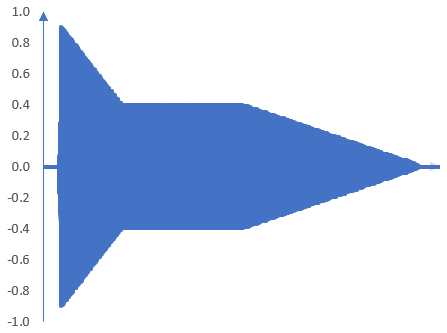The vertical axis is the peak gain. The horizontal axis is the time. The total signal time is about 9600 samples or 1.2 seconds.

The Hilbert transform and the amplitude envelope

I use a Hilbert transform to detect the amplitude envelope of this signal. (We know the amplitude envelope of this signal, but we do not know the amplitude envelopes of most signals that we want to compress. We use this signal to test our computation of amplitude envelopes). The Hilbert transform of length 200 samples is

$$\frac{2}{200} \frac{sin^2(\frac{\pi}{2}(k-\frac{200}{2}))}{tan(\frac{\pi}{200}(k-\frac{200}{2}))}$$

where k are the samples. There are two tricks to applying this Hilbert transform correctly.

• Since we are going to use it in a convolution, it must be inverted in time.
• The amplitude envelope will be computed from the root of the sum of the squared values of the signal after the Hilbert transform and the original signal. Since this is a Hilbert transform of even length, the original signal must be delayed by exactly 200/2 + 1 samples.

The envelope is as expected. It is shown here.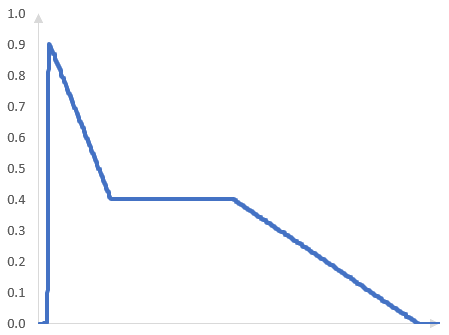If the envelope is not computed correctly, it will look odd as in the following picture.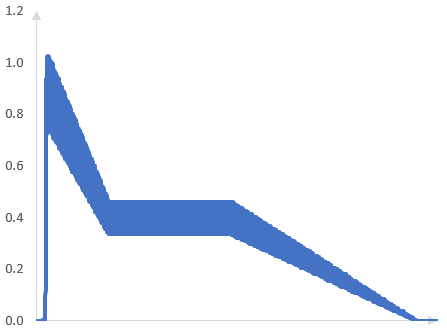A single threshold compressor

A single threshold is easy. The following graph shows the amplitude envelope of the signal after a compressor with a threshold at -5 dB, attack of 1 ms, release of 300 ms, and compression ratio above the threshold of 4:1 (there is no compression or expansion below the threshold).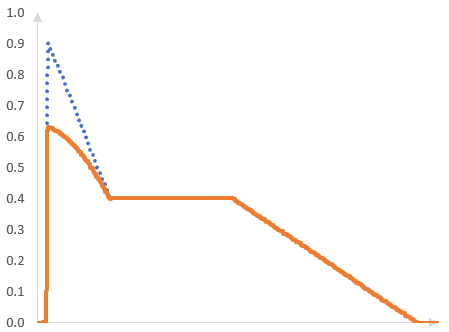A multi threshold compressor

The following graph shows the relationship between the input amplitude and output amplitude of a signal going through a two-threshold compressor. The input amplitude is measured along the horizontal axis and in decibels. The output amplitude, also in decibels, is measured along the vertical axis.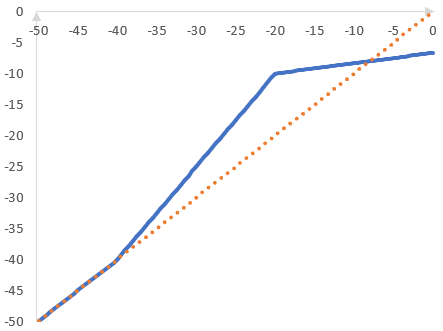In this specific compressor, there is no compression or expansion if the amplitude of the incoming signal is below -40 dB (the first threshold). The signal is expanded with a ratio of 1:1.5 between -40 dB and -20 dB (the second threshold). The signal is compressed with a ratio of 6:1 above -20 dB.

In this graph, the dotted diagonal line is the line for which the output amplitude is always the same as the input amplitude. This line represents no compression or expansion (compression ratio of 1:1). The expansion between -40 dB and -20 dB is shown by the middle segment of the compressor line, which is steeper than the dotted line. The compression above -20 dB is shown by the right segment of the compressor line, which is less steep than the dotted line.

The trick to multi threshold compressors is understanding that the gain applied to the signal above one of the thresholds does not depend only on that threshold and the compression / expansion ratio belonging to that threshold. It also depends on what happens at lower thresholds.

For example, at -15 dB input amplitude on the horizontal axis, the compressor will:

• Take the first threshold of -40 dB. There is no compression or expansion up to this threshold.
• Because of the expansion above -40 dB, but only up to -20 dB, expand that portion of the input gain by adding gain of (-20 – (-40)) / (1 / 1.5) = 30 dB
• Because of the compression above -20 dB, compress the portion of the input gain above -20 dB and will add gain of (-15 – (-20)) / (6 / 1) = 0.833 dB.

The result is -40 + 30 + 0.833 = -9.167 dB. If the input amplitude of the signal is -15 dB, then the output amplitude of the signal is -9.167 dB.

The result is not the same as that of a single-threshold compressor. Take a compressor with a single threshold at -20 dB and compression ratio of 6:1. For this compressor, if the input amplitude is -15 dB, then the output amplitude will be -20 + (-15 - (-20)) * 1 / 6 = -19.167 dB. There is a big difference between this compressor and the two- threshold compressor (at least at -15 dB) and this difference is solely due to the what happens at the lower threshold in the two-threshold compressor.

Thus, we cannot compute what happens above the highest threshold without knowing what happens at the lower thresholds. In the two-threshold compressor, if we simply compress the signal when it is above -20 dB, without considering the expansion below -20 dB, we will have a graph with a discontinuity at -20 dB, such as this one.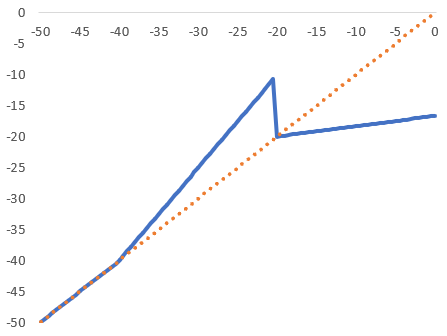This is not very productive. If we apply this type of compressor to a signal, there will be large jumps in the signal amplitude after the compressor.

Amplitude envelope after the two-threshold compressor

The amplitude envelope of the signal after the two-threshold compressor is as follows. The exact amplitude envelope depends, of course, on the compressor gain and attack, but that is a topic of another post.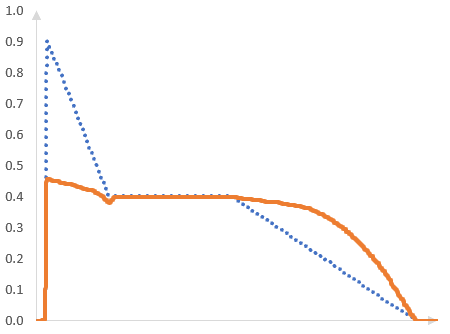authors: mic

Filtered HTML

• Freelinking helps you easily create HTML links. Links take the form of [[indicator:target|Title]]. By default (no indicator): Click to view a local node.